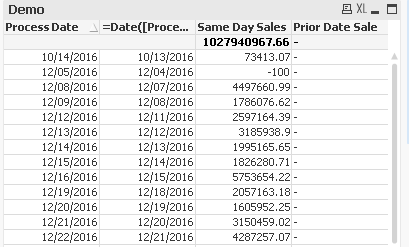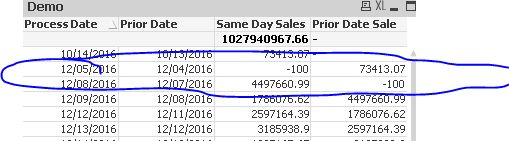# New to QlikView

Discussion board where members can get started with QlikView.

Highlighted
Contributor III

## How to calculate previous Date 's sum(Sales)?

Hi,

I am using the below table:

[Data]:
load * inline [
DateData, Sales
12/11/2017, 2012
12/10/2017, 2015
12/09/2017, 2016
12/08/2017, 2017
12/07/2017, 2018
]
;

and use a straight table:

and use DateData as dimension and Sum({\$<[Process Date] = {"\$(date(only([Process Date]-1)))"}>}[Sales Posting Amount USD]) as expression. But I get '0' for all fields.

Thanks,

Sandip

Tags (1)
1 Solution

Accepted SolutionsMVP

## Re: How to calculate previous Date 's sum(Sales)?

Check if this looks good

13 RepliesMVP

## Re: How to calculate previous Date 's sum(Sales)?

May be try

Above(Sum({<DateData>}Sales)) * Avg(1)

or use The As-Of TableMVP

## Re: How to calculate previous Date 's sum(Sales)?

Here is a sample attachedHonored Contributor

## Re: How to calculate previous Date 's sum(Sales)?

Hi,

May be expression  Above(Sum (Sales))?MVP

## Re: How to calculate previous Date 's sum(Sales)?

AsOfTable example attached

Contributor III

## Re: How to calculate previous Date 's sum(Sales)?

Hi,

I just attached my sample qvw file. Please can you edit the 'Demo' straight table chart. Currently it does not show any data for

'Prior Date Sale' column's value under it.Thanks,

SandipMVP

## Re: How to calculate previous Date 's sum(Sales)?

Since you used two dimension, you needed to add Total after Above

Above(TOTAL Sum({<[Process Date]>}[Sales Posting Amount USD])) * Avg(1)

Contributor III

## Re: How to calculate previous Date 's sum(Sales)?

Hi,

it looks ok. But I need to implement the logic as : if the previous day's value is null or not exists in data model, then it will be null, but you can see that for Prior Date: 12/07/2016 , it is not present in the data model, but the prior date sale value is coming for 12/08/2017. SO it should be null or blank value. So instead of showing -100 it should show only 0 in the table.

So the logic should be if a date's prior date is not present in the data, it will show null or 0 prior date sale's column value.Thanks,

SandipMVP

## Re: How to calculate previous Date 's sum(Sales)?

Then it would be best to use The As-Of Table approach here

Contributor III

## Re: How to calculate previous Date 's sum(Sales)?

Hi,

I have seen it. But I did not understand how to implement it in my case.

So I am requesting you if you can implement it in my sample QVW document.

Thanks,

Sandip